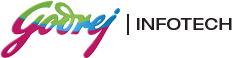# Placement Papers - GodrejGodrej Infotech - K.J Somaiya College, Mumbai
Posted by :
Amit pandey
(395)
Criteria -55% and above no live kt.

10 question in 1 hour difficulty level is medium.
the very first page is full of instructions n rules(3)
The test is known as box test 2D
10,5 ,7 in box1,box2 , box 3.get 12 in box 1 U have to write the box no in the brackets
and 15 in box3.use add only.no of steps reqd =3. along with d operation used.
box-1 box-2 box-3 Result

Example 1: 10 5 7 box no. box no.
Step1 5+7=12 5 7  +  = 
Step 2: 12 5 5+5=10  +  = 
Step 3: 12 5 10+5=15  +  = 

Rules:
1. n+m=n or m where n,m are box no\'s.(if in the final step u get it in this formâ€¦UR ANSWER IS RIGHT)n*m=n or m
2. don\'t remember..something related to subtraction I guess..
3. n/m=dividend..ignore the remainder
(if 9.9..take 9..donot round off)
Note:
U have to get the values in specified no. of steps given in each problem.if u get it in less no of steps then be sure U R GOING WRONG
SOMEwhere.n if u exceed the no of steps as mentioned in d problem then be ready for 25%negative marking(though they have giv
Moreover,even if u get d correct value in only one of the boxes... ur score is zero for that particular problem.(consider the above
example:if u get 12 in box 1 but 25 in box 3 then u will not gain ne mark..i.e,no marks for half right answer..no marks for d steps.).each problem ,they will mention the no.of steps as well as the operation .

Question asked in box test are as given below

1) Box1 contains Son\'s current age is 13 and box2 contains fathers current age is 58 box3 contain sum of father and son six yrs back.
bring father\'s age 13yrs before son was born in box 1 and sum of father and son after 7yrs.

2) Box 1 cotain odd value X or Y the box 2 =X i.e 7 or 12 box 3= Y has value 19 if X is 7 or 31 if x is 12 get even value X or Y Use any operator steps allowed 3.

3)box1: p=cost of a dozen bag
box2: 84(duty on 12bags)
box3: 7(num of bag purchased by customer)
bring cost + duty of 7 bags in box 1

4)-1,0 or 2 in box1 and -A in box 2, A,(A-1),(A+1) box3
get A(A-1)(A-1)A in box-2 and in 3 steps,Use all operations.

5)Another example is AB in box1, AC in box2,BC in box3 then
get 2C(B+A)-B(C-3A) in box1 in 4 steps or 2A(B+C)+AC-2C(A-B) in 3 steps

6)69,70,71 18,19,20 27,28,29 get -3,-4,-5 in box 2 ...use ne/all operations..4 steps

7)there is a question like there are 3 living animal human,dog and snake
in box 1-62 the total no of eyes of living animals
box2-40 no of legs of living animals
box3 -15 no of snakes
put the no of dogs in box 1and no of human in box 2
using multiply and diivde in 3 steps.

8)t is the total number of sun glass sold which has c number of cartons and l loose glasses. Each cartons contains 27
glasses. If each sale of carton, 3rs discount is awarded on each glass. Each glass cost rs9.
Box1- total number of glass sold
box2- Cost of each glass
box3-discount on each glass
using any operation in 6 steps, bring total discount and total selling price in box2 and box3 respectivily

9)Box1 box2 box3
13  11   7
using ony addition and subtraction bring 19 in box1 and 30 in box3.

10)it is same as ques .9

ALL THE BEST